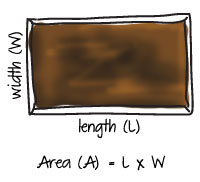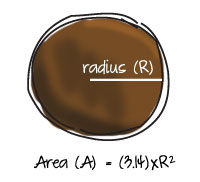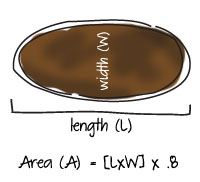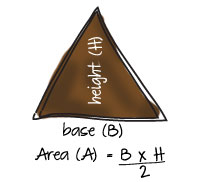100% Grow Guarantee!

# Garden Area Calculator

## 1Determine How Many Square Feet You Have:

To determine how many square feet you have, you will use the formulas listed below to find area (A) - this mathematical formula will be different based on the shape of your garden. First, you will need to go outside and measure your garden bed. You will need to have the proper measurements handy, such as width (W), length (L), radius (R), base (B), or height (H).### Rectangular/Square Garden

A rectangle and square both have four right angles. The area of a rectangle or square is found by multiplying the length (L) by the width (W).### Circular Garden

A circle is a closed curve of which every point on the edge of the curve is the same distance from the center point. The area of a circle is the radius squared (R)x(R) multiplied by pi (~3.14). The radius is equal to one-half the diameter of the circle.### Oval Shaped Garden

An oval has an elliptical, or egg-like, shape. The area of an oval is the length (L) multiplied by the width (W), multiplied by 0.8.### Triangular Garden

A triangle is a polygon with three sides. The area of a triangle is one-half the base (B) multiplied by the height (H).### Irregularly Shaped Garden

To find the area of a large irregular shape, divide it into a series of smaller units equally spaced along a measured line. This method will calculate the area to within 5%.

1. Determine the length line. This is the longest axis of the shape as shown in the image from point A to point B.
2. Mark width lines along the A-B line into several segments of equal width. To ensure accuracy, use as many width lines as possible. The more irregular the shape, the more width lines you will need.
3. Measure the width at each width line. In this example, there are 5 width lines: C, D, E, F and G.
4. Calculate the area by adding all of the width lines together and multiplying the total by the distance between each line (C+D+E+F+G) x Segment Width.

## 2Decide How Many Bulbs You Need

Now that you know how many square feet you have, you will now determine how many bulbs you need to fill it! Use the table below to calculate how many bulbs you need per square feet. Multilply your square feet (sq ft) by the number of bulbs (# of bulbs) for your total number of bulbs needed. For example: If your garden is 10 square feet and you want to fill it with tulips, you will multiply: 10 (sq feet) x 5 (# of bulbs) to equal 50.

Type of Bulb Bulbs Per Square Foot
Tulips 5
Daffodils 4-5
Bearded Iris 1
Hyacinths 3-4
Dutch Iris 6-8
Allium (large/small) 1-2/8-12
Fritillaria 1-2
Muscari 14-18
Scilla 15-16

## Planting Information

### Holiday Bulbs

Garden Area Calculator